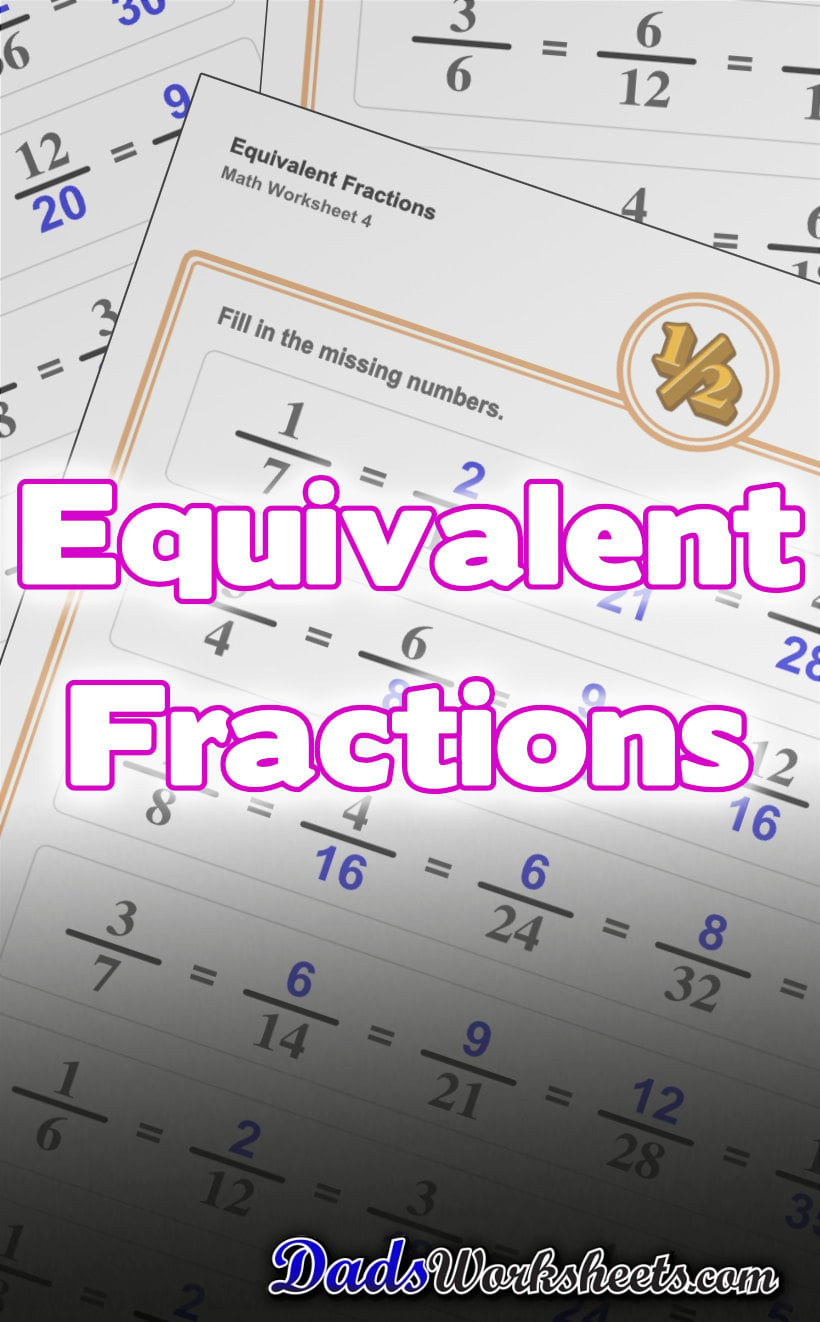# Equivalent Fractions Worksheets

Equivalent fractions are on of the earliest concepts students need to learn before proceeding to reducing fractions, or when changing fractions to have a common denominator when adding and subtracting fractions with different denominators. These worksheets start with familiar fractions like halves and quarters and proceed through common fractions with larger denominators. Finding equivalent fractions is a great topic to introduce in 3rd or 4th grade before moving on to adding fractions or subtracting fractions.

If you really need an amazing tool for visualizing equivalent fractions, be sure to check out this fraction chart which shows families of equivalent fractions in an elegant way along with their decimal equivalents.

## Need More Fraction Worksheets?

After mastering these equivalent fractions worksheets, are you looking for more fraction practice? Check out these fraction resources for more tools appropriate for your 3rd, 4th or 5th grade students:

## Check Out These Equivalent Fraction Charts!

These fraction charts show where specific groups of equivalent fractions land on the number line with their decimal equivalents. Ready to print for free as a student notebook fraction anchor chart, or order it as a beautiful classroom poster!

Fraction Chart